Piecewise spectrally band-pass for compressive coded aperture spectral imaging
Key Laboratory of Computational Optical Imaging Technology, Academy of Opto-electronics, Chinese Academy of Sciences, Beijing 100094, China

Corresponding author. E-mail: xiangli@aoe.ac.cn

*Project supported by the National Natural Science Foundation for Distinguished Young Scholars of China (Grant No. 61225024) and the National High Technology Research and Development Program of China (Grant No. 2011AA7012022).

Abstract

Coded aperture snapshot spectral imaging (CASSI) has been discussed in recent years. It has the remarkable advantages of high optical throughput, snapshot imaging, etc. The entire spatial-spectral data-cube can be reconstructed with just a single two-dimensional (2D) compressive sensing measurement. On the other hand, for less spectrally sparse scenes, the insufficiency of sparse sampling and aliasing in spatial-spectral images reduce the accuracy of reconstructed three-dimensional (3D) spectral cube. To solve this problem, this paper extends the improved CASSI. A band-pass filter array is mounted on the coded mask, and then the first image plane is divided into some continuous spectral sub-band areas. The entire 3D spectral cube could be captured by the relative movement between the object and the instrument. The principle analysis and imaging simulation are presented. Compared with peak signal-to-noise ratio (PSNR) and the information entropy of the reconstructed images at different numbers of spectral sub-band areas, the reconstructed 3D spectral cube reveals an observable improvement in the reconstruction fidelity, with an increase in the number of the sub-bands and a simultaneous decrease in the number of spectral channels of each sub-band.

PACS: 07.60.–j; 42.30.Wb; 29.30.–h
Keyword: coded aperture; spectral imaging; compressive sensing; information reconstruction
1. Introduction

As a significant research direction in the field of optical remote sensing, imaging spectrometry has many applications including remote sensing,  geology,  biomedicine,  and the ocean environment. In the early years, priority was given to conventional dispersive imaging spectrometry.[5, 6] With the development of technology, new types of imaging spectrometers appear constantly, and play important roles in different application fields.

Recently, a novel imaging spectrometry called compressive coded aperture spectral imaging, also known as coded aperture snapshot spectral imaging (CASSI),  has been put forward. Based on the conventional imaging spectrometry, a coded mask, which modulates and compresses the three-dimensional (3D) spatial-spectral data-cube about the scene, is introduced appropriately in the light path. The 3D spatial-spectral information about a scene of interest is first encoded and captured with one snapshot at the two-dimensional (2D) detector array. Compressed sensing (CS)[10, 11] theory is then used to reconstruct the 3D data-cube from the 2D measurement. Compared with the conventional imaging spectrometry, CASSI eliminates the disadvantages of low light throughput and temporal scanning, greatly reduces the quantity of original data, and alleviates the pressure of the storage and transmission of information.

According to CS theory, when the number of sampling points does not satisfy the Nyquist sampling theory, the sufficient condition that the signal can still be reconstructed precisely is that the observed signal is possessed of sparsity. In the current CASSI system, the process from 3D spatial-spectral information to 2D compressed sensing imaging is realized, but sparse sampling is only taken into account in the spatial dimension while aliasing and compressing are considered in the spectral dimension. As a result, the fidelity of the reconstruction is very low. In this paper, the imaging process of CASSI is described and the key factors which affect the reconstruction of the 3D spatial-spectral information are analyzed. An improved proposition is presented based on the concept of CASSI. Through computer simulation and comparison, the efficiency of this betterment is verified.

2. A brief introduction to CASSI
2.1. Imaging model of CASSI

CASSI integrates spectral imaging, numerical computation method, compressed sensing, and other disciplines. Through a combination of optical and computational methods, the field of view (FOV) is expanded from a line in the conventional imaging spectrometer to a plane which greatly increases the light throughput. Through a combination of optics and compressed sensing, spatial-spectral imaging information of a 3D data-cube is acquired with just a single 2D measurement of the coded and spectrally dispersed source field. The quantity of original data acquired by the instrument is greatly reduced and the stability of the instrument is remarkably improved. The basic principle of CASSI is shown in Fig.  1.

 Figure Option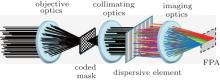Fig.  1. Schematic diagram of CASSI.

As shown in Fig.  1, the incident light propagates through the objective optics to the coded mask considered as a specific modulator, and then the modulated signal passes through the collimating optics to the dispersive element for coded and spectrally dispersed information, and finally, after the imaging optics, the coded and spectrally dispersed signal is received by the detector to obtain compressed sensing spatial-spectral aliasing images. Assuming that the optical system is a 4f system, the system in Fig.  1 can be equivalently simplified as shown in Fig.  2.

 Figure Option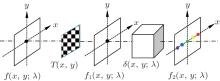Fig.  2. Equivalent imaging model of CASSI.

Let T(x, y) be the transmission function printed on the coded mask. The spectral density entering into the system is denoted as f(x, y; λ ). After being modulated by the coded aperture, the spectral density arriving at the collimating optics is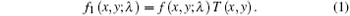Assume that the dispersion only occurs along the x direction and the dispersive element is provided with dispersion coefficient β (λ ) and central wavelength λ c. After propagating through the coded mask, the collimating optics, the dispersive element, and the imaging optics, the light has the spectral density at the focal plane array (FPA):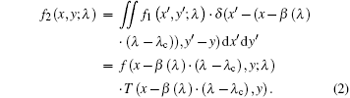Particularly, the measurement at the detector array is an intensity image of the incident object rather than the spectral density. The obtained image on the detector can be represented as an integration process of the spectral density in the range of wavelengths at the corresponding point,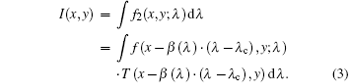In Eq.  (3), we can say that CASSI is also a “ point to point” imaging technique, and the information acquired by the detector is the superposition of the signals of different wavelengths and spatial points. Therefore, when adopting the same optical system, the energy efficiency of CASSI is close to the ordinary camera and the detecting sensitivity is higher than the spectral imager with a slit in the light path.

2.2. Spatial-spectral reconstructing process of CASSI

The reconstruction of CASSI is to invert the 3D spatial-spectral information about the object scene from the aliasing 2D image. As the quantity of the measurements on the detector is much less than the number of spatial points multiplied by the number of spectral channels, the system is under-determined. This under-determined problem can be resolved by CS theory.

In the CS theory, as long as the signal is compressible (or sparsely represented), then it can be reconstructed with high probability at a sampling frequency much lower than the standard Nyquist sampling frequency. The two main principles of CS theory are sparsity and incoherence. The former pertains to the signals of interest, and the latter pertains to the sensing modality. A signal fRN is K-sparse on an orthonormal basis Γ if f can be represented as a linear combination of K vectors of Γ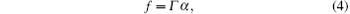with KN and at most K no-zero components in α .

If we use an observation basis HM× N (MN) incoherent with Γ to observe the coefficient vector, after linear transformation, the observing collection ω M× 1 is acquired. Then f can be reconstructed with high probability from ω M× 1 using the optimization method. The mathematical model of the CS theory is shown in Fig.  3.

 Figure Option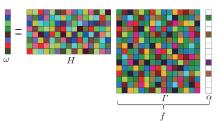Fig.  3. Mathematic model of CS theory.

For the reconstructing process of compressed sensing information, the essence of the final analytic function is to solve the nonlinear optimization problem under certain conditions. That is,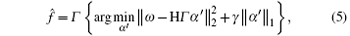where γ is a regularization parameter to adjust the sparsity of the data. In the case that the observation matrix is known, the key work to solve Eq.  (5) is to select the appropriate sparse projection matrix, yielding the optimal α .

3. Analysis of CASSI

In general, the imaging chain of CASSI includes two processes, i.e., 2D compressed sensing imaging of 3D spatial-spectral information, which is the process of information acquisition; and 3D spatial-spectral data-cube reconstructed from a 2D compressed sensing image, which is the process of information reconstruction.

For the process of information acquisition, choosing the coded function (the observation matrix), which will affect the accuracy of reconstruction, is especially significant. There are already some researches of the design and selection of the coded function, including the form of continuous orthogonal function, discrete S-matrix codes and random function. However, in most cases, the correlation length of the observed object is usually very small. Random function is the optimal form of the coded function.[17, 18]

For the process of information reconstruction, compressed sensing reconstruction algorithms are mainly used. Currently, there are varieties of reconstruction algorithms, including matching pursuit (MP),  orthogonal matching pursuit (OMP),  gradient projection for sparse reconstruction (GPSR),  and two-step iterative shrinkage/threshold (TwIST). The above algorithms behave well in compressed sensing imaging of 2D sparse sampling. However, the reconstructed result is not perfectly compellent in the cases of compressed sensing and aliasing of 3D spectral images. As can be seen in Ref.  , the reconstructed images appear distortional in some way. There are two reasons for this phenomenon. First, the solution reconstructed by the TwIST algorithm is not optimal. Second, the quantity of information acquired by the detector from single sampling does not satisfy the reconstruction condition with high probability. In CASSI, the latter is the main reason, because the core of reconstruction condition lies on the restricted isometry property (RIP) which accounts for an optimal fraction among the quantity of sparse sampling, observation data and the reconstructed data. That is,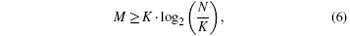where K is the sparsity of the signal and M is the size of the measured signal. In other words, to reconstruct a signal with sparsity K completely, the quantity of the measurements M must meet the above condition. For instance, if the input data-cube is composed of 512× 512 pixels and 33 spectral channels, the quantity of the FPA measurements will be 512× 544. Supposing the spatial dimension and the spectral dimension are also K-sparse, an equation can be obtained as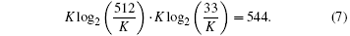Through iterative computation, K is approximately 6. Apparently, whether in spatial dimension or in spectral dimension, it is extremely difficult to truly express the original information with 6 sparse sampling points. Therefore, merely through single exposure sampling in CASSI, it is insufficient to reconstruct the 3D data-cube with high probability.

Furthermore, in the CASSI system, sparse sampling only occurs in the spatial dimension but aliasing sampling appears in the spectral dimension. Since spatial-spectral aliasing only appears in the dispersive dimension, we select a slice in the y-dimension to describe sparse sampling and spatial-spectral aliasing as shown in Fig.  4. The intensity of each pixel at the focal plane array is the aliasing information of the spatial sparse sampling information expanded and migrated along the spectral dimension. For example, the intensity of e5 can be expressed as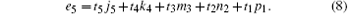Only e5 and t1t5 are known in Eq.  (8). Considering the pixels e1 · · · e9, we can structure a system of linear equations as follows: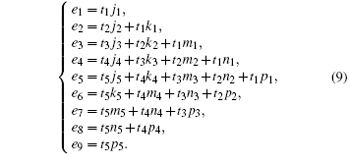Figure Option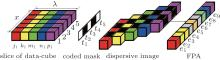Fig.  4. Discrete model of CASSI.

As shown in Eq.  (9), the system is underdetermined. Without any constraint, it is unable to obtain the accurate solution. Thus, in CASSI, the inversion of 3D spatial-spectral data-cube is not only the reconstruction of the sparse sampling information, but also the process of resolving aliasing spatial-spectral information. To ensure the correctness of the solution, the spectral cube should be possessed of high sparsity within the set of solutions. To improve the accuracy of the solution, the amount of sampling information should be increased.

4. Analysis and simulation of improved CASSI

According to the above analysis, only by a single exposure is it insufficient to reconstruct the 3D data-cube accurately. So one proposed multi-frame imaging to improve the accuracy of the solution,  and a higher-order computational model leading to image reconstructions less dependent on calibration also improves the image quality reconstruction of the underlying signals. It is worth noting that the increase of exposure shots only increases the amount of sampling information in the spatial dimension and does not pay significant attention to the reconstruction of spectral aliasing. Because of the long sampling time, the advantage of snapshot imaging is lost. In addition, there must exist a moving element to change the coded aperture in different shots, which will weaken the stability of the system.

Because the CASSI system usually works in a wide spectral range and the spectral information does not commonly meet the high sparsity condition, it is difficult to reconstruct the data-cube accurately. Thus we look for an optimization scheme which meets high sparsity condition in the set of solutions and operates finely in a wide spectral range. To ensure high sparsity in the spectral dimension, we propose the improved system through the method of band division.

In the improved system, the mask in the first image plane is not only a coded function but also a spectrally band-pass filter array. The first image plane is divided into several continuous segments. In order to enhance continuity of the spectral dimension, there is an overlapping channel between adjacent sub-band areas as shown in Fig.  5. Each sub-band is continuous in the spectral dimension and spatial dimension, but not complete in the 3D space.

 Figure Option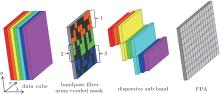Fig.  5. Image model of improved CASSI.

Assuming that the object scene {fijh} has M × N pixels and L spectral channels, the coded mask {tpq} has M × N elements, the full-band is segmented into Q sub-bands and each sub-band consists of P spectral channels. Between adjacent sub-band areas, an overlapping channel is considered. P, Q, and L are related by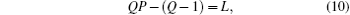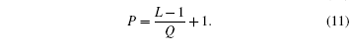The measurement at the focal plane array can be expressed as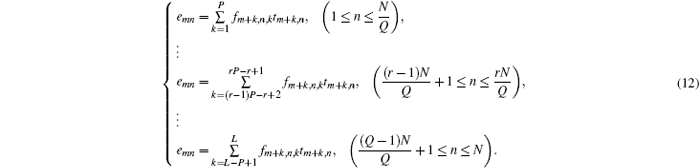Equation  (12) can also be written as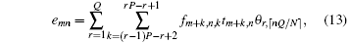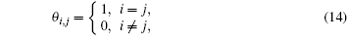where ⌈ χ ⌉ rounds the elements of χ to the nearest integers greater than or equal to χ . Therefore, the scanning of the field of view (FOV) is required to acquire the complete 3D data-cube. Compared with multi-frame imaging through shifting the coded mask in traditional CASSI, the sparsity of the sub-band is obviously improved and multiple shots are achieved along with the relative movement of the instrument and the object scene. There is no need to increase movement components inside the instrument. So it has the advantage of high stability and good adaptability to the environment.

We still take a single slice of a spectral cube for example, and suppose that the number of spectral channels changes from five in CASSI to three as shown in Fig.  6. Like the traditional CASSI, the intensity of each pixel at the detector array is composed of a coded linear combination of the spectral information from the respective data cube slice. Due to the spectral band range being reduced, the system of linear equations can be written as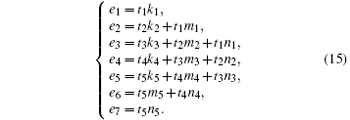Figure Option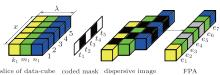Fig.  6. Discrete model of improved CASSI.

In Eq.  (15), with the decrease of the number of spectral channels, the numbers of unknowns and equations are also reduced. The decrement of unknowns is in accordance with the number of the pixels in single-band, multiplied by the number of the reduced channels, which is quicker than the variation of the number of equations. At the same time, the sparsity in the spectral dimension is improved. Therefore, the accuracy of the solution under this condition is higher than the CASSI system.

In order to verify the effectiveness of improvement, some imaging simulations are carried out. The original source is generated by free data. For computational regions, our source is limited to one of the spatial regions (512× 512 pixels, and 33 spectral channels). An intensity image of the original source is shown in Fig.  7. The coded function also consists of the discrete random 512× 512 binary element pattern.

 Figure OptionFig.  7. Intensity image of source.

According to Eq.  (11), L = 33, the number of the sub-bands (Q) and the number of spectral channels of each sub-band (P) could be in different combinations. Q = 1, P = 33 (the traditional CASSI); Q = 2, P = 17; Q = 4, P = 9; Q = 8, P = 5 are seriatim implemented in the same settings. The TwIST algorithm is used to reconstruct the spectral cube from continuous pushbroom imaging results. The intensity images of the reconstructions are shown in Fig.  8.

 Figure Option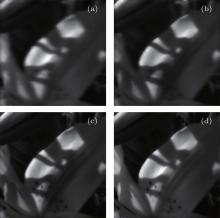Fig.  8. Intensity images of the reconstructions at (a) Q = 1, P = 33; (b) Q = 2, P = 17; (c) Q = 4, P = 9; (d) Q = 8, P = 5.

In order to quantitatively analyze the advantage of the improved system, the peak signal-to-noise ratio (PSNR) and information entropy are used to evaluate the fidelity of reconstructed data-cube as shown in Table  1. Information entropy is defined as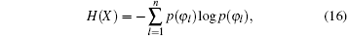where p(φ l) is the normalized frequency of the l-th gray value in image φ l.Table 1. PSNR and information entropies of the reconstructions with different numbers of sub-bands.

Figure  9 shows PSNR and entropy change of the reconstructed data cubes each as a function of the number of sub-bands. The benefit achieved by the improved system is quantitatively remarkable by comparing with the PSNR and the entropy change of the reconstructed data cubes. The improvement of PSNR approaches to 5.3  dB and information entropy is also closer to the original when the number of sub-bands is 8. As can be seen in Ref.  , the reconstruction can achieve a 4-dB improvement. In order to obtain multi-frame images, the system must stare at the object for a long sampling time, so it is favorable for capturing the static scene. However, in airborne and spaceborne applications, there is usually relative movement between the instrument and the object. The advantage of the system in this paper will emerge.

 Figure Option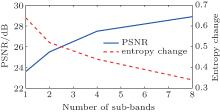Fig.  9. PSNR and entropy changes of the reconstructed images with the number of sub-bands.

5. Conclusions

CASSI fully demonstrates the advantages of a multidisciplinary fusion, and makes up for the shortcomings of the conventional imaging spectrometry. In this paper, an improved system is presented, which commendably accounts for the degree of spatial-spectral aliasing image, and improves the spectral sparsity within the reconstructed spectral channels. The quality of the reconstructed data is noticeable with higher PSNR and closer information entropy than the traditional CASSI. Since there does not exist any moving element in the system, its stability and reliability are enhanced. This amelioration makes CASSI more suitable for real applications such as aerospace remote sensing. Moreover, the TwIST algorithm generates image smoothing and debases the image resolution. The reconstructions are not really optimal. Our future research will focus on more accurate reconstructions through appropriately changing reconstructed basis. However, there are also some theoretical and technical issues which need to be addressed. First, there is no quantitative evaluation criterion about the sparsity of information, which determines the minimal number of observations to reconstruct the compressive information. The coded function also needs to be more optimal for high reconstruction fidelity.

Reference
 1 Smith W L, Zhou D K, Harrison F W, Revercomb H E, Larar A M, Huang H L and Huang B 2001 Proc. SPIE 4151 94 [Cited within:1] 2 Clark R N, King T V V, Klejwa M, Swayze G A and Vergo N 1990 J. Geophys. Res. 95 12653 DOI:10.1029/JB095iB08p12653 [Cited within:1] 3 Kang U, Berezin V B, Papayan G V, Petrishchev N N and Galagudza M M 2013 J. Opt. Technol. 80 40 DOI:10.1364/JOT.80.000040 [Cited within:1] 4 Yu K and Hu C M 2013 J. Appl. Rem. Sens. 7 073589 [Cited within:1] 5 Xiang Li B, Yuan Y and Lü Q B 2009 Acta Phys. Sin. 58 5399(in Chinese) [Cited within:1] 6 Nan Y B, Tang Y, Zhang L J, Chang Y E and Cheng T A 2014 Acta Phys. Sin. 63 010701(in Chinese) [Cited within:1] 7 Wagadarikar A, John R, Willett R and Brady D 2008 Appl. Opt. 47 B44 DOI:10.1364/AO.47.000B44 [Cited within:1] 8 Wagadarikar A A, Pitsianis N P, Sun X B and Brady D J 2008 Proc. SPIE 7076 707602 [Cited within:1] 9 Arce G R, Brady D J, Carin L, Arguello H and Kittle D S 2014 IEEE Signal Process. Mag. 31 105 DOI:10.1109/MSP.2013.2278763 [Cited within:1] 10 Donoho D L 2006 IEEE Trans. Inform. Theory 52 1289 DOI:10.1109/TIT.2006.871582 [Cited within:1] 11 Cand ès E J and Wakin M B 2008 IEEE Signal Process. Mag. 25 21 DOI:10.1109/MSP.2007.914731 [Cited within:1] 12 Ning F L, He B J and Wei J 2013 Acta Phys. Sin. 62 174212(in Chinese) [Cited within:1] 13 Kim S J, Koh K, Lustig M, Boyd S and Gorinevsky D 2007 IEEE J. Sel. Topics Signal Process. 1 606 DOI:10.1109/JSTSP.2007.910971 [Cited within:1] 14 Cand ‘es E J, Romberg J and Tao T 2006 IEEE Trans. Inform. Theory 52 489 DOI:10.1109/TIT.2005.862083 [Cited within:1] 15 Wagadarikar A A, Gehm M E and Brady D J 2007 Appl. Opt. 46 4932 DOI:10.1364/AO.46.004932 [Cited within:1] 16 Arguello H and Arce G R 2011 J. Opt. Soc. Am. A 28 2400 DOI:10.1364/JOSAA.28.002400 [Cited within:1] 17 Meng J, Yin W T, Li H S, Hossain E and Han Z 2011 IEEE J. Sel. Areas Commun. 29 327 DOI:10.1109/JSAC.2011.110206 [Cited within:1] 18 Sun B and Zhang J J 2011 Acta Phys. Sin. 60 110701(in Chinese) [Cited within:1] 19 Mallat S and Zhang Z 1993 IEEE Trans. Signal Process. 41 3397 DOI:10.1109/78.258082 [Cited within:1] 20 Davenport M A and Wakin M B 2010 IEEE Trans. Inform. Theory 56 4395 DOI:10.1109/TIT.2010.2054653 [Cited within:1] 21 Figueiredo M, Nowak R D and Wright S J 2007 IEEE Sel. Top. Signal Process. 1 586 DOI:10.1109/JSTSP.2007.910281 [Cited within:1] 22 Bioucas-Dias J M and Figueiredo M A T 2007 IEEE Trans. Image Process. 16 2992 DOI:10.1109/TIP.2007.909319 [Cited within:1] 23 Cand ès E J 2008 C. R. Math. Acad. Sci. Paris 346 589 DOI:10.1016/j.crma.2008.03.014 [Cited within:1] 24 Kittle D, Choi K, Wagadarikar A A and Brady D J 2010 Appl. Opt. 49 6824 DOI:10.1364/AO.49.006824 [Cited within:1] 25 Arguello H, Rueda H, Wu Y H, Prather D W and Arce G R 2013 Appl. Opt. 52 D12 DOI:10.1364/AO.52.000D12 [Cited within:2] 26 http://personalpages.manchester.ac.uk/staff/david.foster/Hyperspectral_images_of_natural_scenes_04.html [Cited within:1] 27 Zhang C T, Ma Q L and Peng H 2010 Acta Phys. Sin. 59 7623(in Chinese) [Cited within:1]